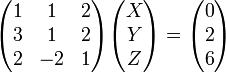# Gaussian elimination

Gaussian elimination, sometimes called simply elimination, is a method in mathematics that is used to solve a system of linear equations. Such sets of equations occur throughout mathematics, physics, and even in the optimization of business practices, such as scheduling of bus routes, airlines, trains, and optimization of profits as a function of supplies and sales. The method can be accomplished using written equations, but is more often simplified using matrix forms of the equations.

Three basic maneuvers are allowed in the Gaussian elimination method:

1. Interchanging any two equations
2. Multiplying both sides of any equation by a non-zero number
3. Adding a multiple of one equation to another equation

## Equation format

Consider the following system of linear equations that must all be satisfied simultaneously:

• (Eq. 1) 1X + 1Y + 2Z = 0
• (Eq. 2) 3X + 1Y + 2Z = 2
• (Eq. 3) 2X − 2Y + 1Z = − 6

Typically, the equation with the simplest form of X is placed on the top of the equation list. Subsequently, variable X is removed from the second or third equation by the addition or subtract of the first equation, or a multiple thereof. Subtracting 3 times equation 1 from equation 2 eliminates X from equation 2, as shown below.

• (Eq. 1) 1X + 1Y + 2Z = 0
• (Eq. 2) 0X − 2Y − 4Z = 2
• (Eq. 3) 2X − 2Y + 1Z = − 6

After removing X from the third equation in a similar fashion, that is subtracting 2 times Eq. 1 from Eq. 2, one obtains

• (Eq. 1) 1X + 1Y + 2Z = 0
• (Eq. 2) 0X − 2Y − 4Z = 2
• (Eq. 3) 0X − 4Y − 1Z = − 6

Once one variable has been removed from all but one equation, and second variable is removed from all but one equation. In this set of equations for example, the variable Y can be removed from equation Eq. 1 by addition of 1/2 Eq. 2 to it, and Y can be removed from Eq. 3 by subtracting twice Eq. 2 from it, resulting in

• (Eq. 1) 1X + 0Y + 0Z = 1
• (Eq. 2) 0X − 2Y − 4Z = 2
• (Eq. 3) 0X + 0Y + 5Z = − 10

Equations 2 & 3 can be simplified by division, yielding the following set of equations

• (Eq. 1) 1X + 0Y + 0Z = 1
• (Eq. 2) 0X − 1Y − 2Z = 1
• (Eq. 3) 0X + 0Y + 1Z = − 2

The last step of elimination entails removing the variable Z from equation 2. Note that Z was removed from equation 1 previously at the time that Y was removed. By adding twice equation 3 to equation 2, and subsequently multiplying equation 2 by −1, one obtains the final solution.

• (Eq. 1) 1X + 0Y + 0Z = 1
• (Eq. 2) 0X + 1Y + 0Z = 3
• (Eq. 3) 0X + 0Y + 1Z = − 2

Thus, the ordered triplet (1, 3, −2) is the simultaneous solution to this set of equations.

• (Eq. 1) X = 1
• (Eq. 2) Y = 3
• (Eq. 3) Z = − 2

## Matrix format

The repeated writing of the variables is time consuming and unnecessary, particularly when the number of equations and variables is greater than 3 or 4. Thus, using a matrix representation is often used. The example shown previously can be written succinctly as$\begin{pmatrix} 1 & 1 & 2 \\ 3 & 1 & 2 \\ 2 & -2 & 1 \end{pmatrix} \begin{pmatrix} X \\ Y \\ Z \end{pmatrix} = \begin{pmatrix} 0 \\ 2 \\ 6 \end{pmatrix}$

and the final solution, shown in echelon form, is$\begin{pmatrix} 1 & 0 & 0 \\ 0 & 1 & 0 \\ 0 & 0 & 1 \end{pmatrix} \begin{pmatrix} X \\ Y \\ Z \end{pmatrix} = \begin{pmatrix} 1 \\ 3 \\ -2 \end{pmatrix}$

The steps used to solve the matrix representation are identical to those shown for the equation format shown above. Only the notation has changed.Some content on this page may previously have appeared on Citizendium.